﻿ 爆炸与冲击问题的大规模高精度计算
«上一篇文章快速检索 高级检索

 力学学报2016, Vol. 48Issue (3): 599-608  DOI: 10.6052/0459-1879-15-3820

### 引用本文 [复制中英文]

[复制中文]
Ma Tianbao, Ren Huilan, Li Jian, Ning Jianguo. LARGE SCALE HIGH PRECISION COMPUTATION FOR EXPLOSION AND IMPACT PROBLEMS[J]. Chinese Journal of Theoretical and Applied Mechanics, 2016, 48(3): 599-608. DOI: 10.6052/0459-1879-15-382.
[复制英文]

### 文章历史

2015-10-20 收稿
2016-01-04 录用
2016-01-13 网络版发表.

1 冲击波强间断问题高分辨率数值模拟的伪弧长方法研究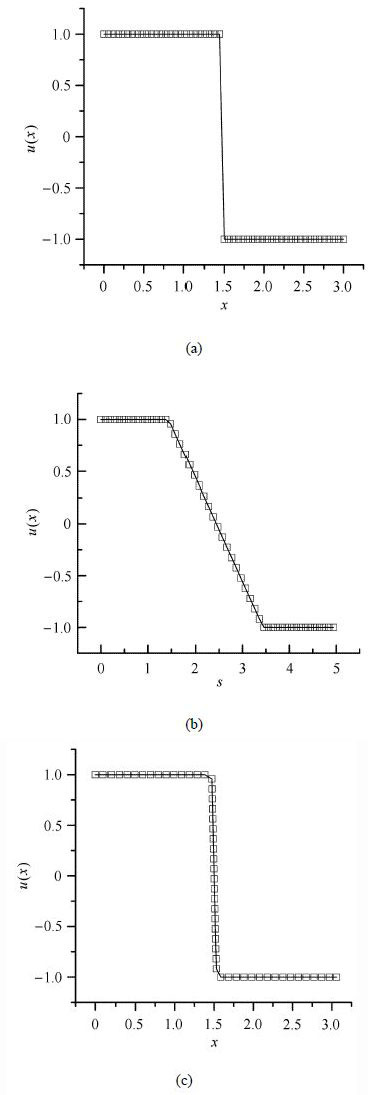图1 应用伪弧长方法物理量空间分布变化过程 Fig.1 Physical quantities space distribution change process with pseudo arc-length method

 $\frac{\partial }{\partial t}w+\frac{\partial }{\partial x}F\left( w \right)=s\left( w \right),t\ge 0$ (1)

 ${{\left( d\xi \right)}^{2}}={{\left( dx \right)}^{2}}+\sum\limits_{i=1}^{n}{{{\lambda }_{i}}}{{\left( d{{w}_{i}} \right)}^{2}}$ (2)

 $M\left( x,t \right)=\sqrt{1+\sum\limits_{i=1}^{n}{{{\lambda }_{i}}{{\left( \nabla w \right)}^{2}}}}$ (3)

 ${{x}_{t}}=\frac{1}{\tau }\frac{\partial }{\partial \xi }(M\frac{\partial x}{\partial \xi })$ (4)

 \begin{align} & |K|\frac{\partial }{\partial t}\bar{w}=-\sum\limits_{l}{h}(w_{K}^{\text{int}(l)},w_{K}^{\text{ext}(l)},n_{K}^{l})|e_{K}^{l}|- \\ & |K|s(\bar{w}) \\ \end{align} (5)

 $\sum\limits_{K}{\left| {{{\tilde{A}}}_{K}} \right|}{{\tilde{w}}_{K}}=\sum\limits_{K}{\left| {{A}_{K}} \right|}{{w}_{K}}$ (6)

 $R\to P,\omega =-\tilde{K}\rho R\exp \left( -\tilde{T}/T \right)$ (7)

 $E=\frac{1}{2}\rho {{u}^{2}}+\frac{p}{\gamma -1}+\rho qR$ (8)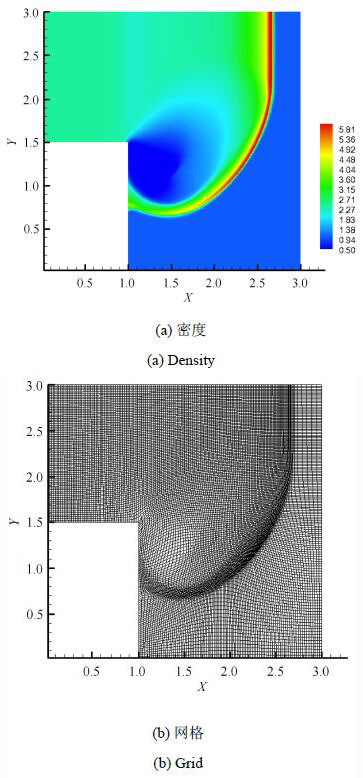图2 $t =0.3$时数值模拟结果 Fig.2 Numerical results at $t = 0.3$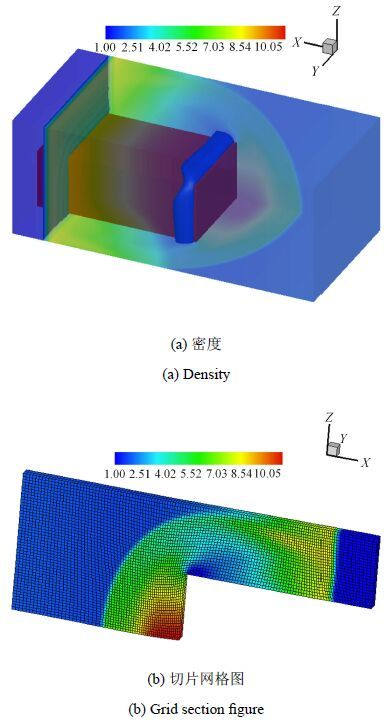图3 $t =0.178$时，数值模拟结果 Fig.3 Numerical results at $t = 0.178$
2 基于附加龙格-库塔方法的高精度气相爆轰数值模拟

 $dU/dt=F(U)=\sum\limits_{\nu =1}^{N}{{{F}^{[\nu ]}}}(U)$ (9)

 $\left. \begin{array}{*{35}{l}} {{U}^{(i)}}={{U}^{n}}+(\Delta t)\sum\limits_{\nu =1}^{N}{\sum\limits_{j=1}^{s}{a_{ij}^{[\nu ]}}}{{F}^{[\nu ]}}({{U}^{(j)}}) \\ {{U}^{n+1}}={{U}^{n}}+(\Delta t)\sum\limits_{\nu =1}^{N}{\sum\limits_{j=1}^{s}{b_{i}^{[\nu ]}}}{{F}^{[\nu ]}}({{U}^{(j)}}) \\ \end{array} \right\}$ (10)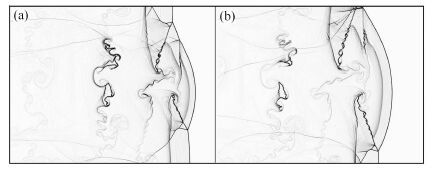图4 两个不同时刻的爆轰波波阵面精细结构 (密度梯度) Fig.4 Detonation wave front fine structure at two different times (density gradient)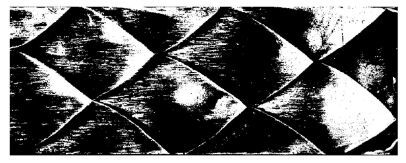图5 实验胞格结构 Fig.5 Experimental cell structures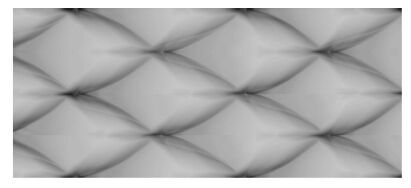图6 数值胞格结构 Fig.6 Numerical cell structures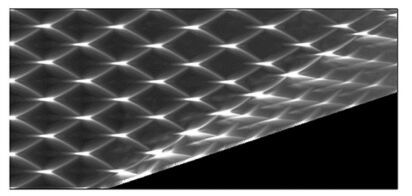图7 爆轰波马赫反射的胞格结构 Fig.7 The cell structure of detonation wave Mach reflection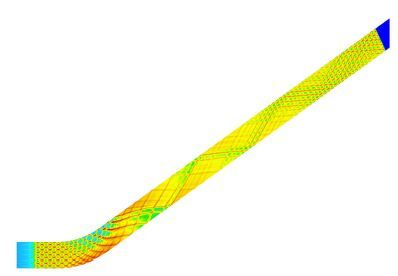图8 爆轰波在30°弯管中传播的胞格结构 Fig.8 The cell structure of detonation wave propagation in 30° bend
3 三维爆炸与冲击问题的大规模并行计算

3.1 子区域间的关联性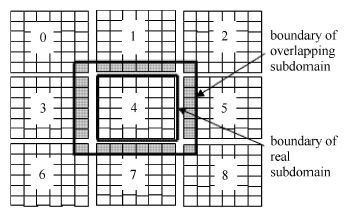图9 重叠子区域 Fig.9 The overlapping subdomain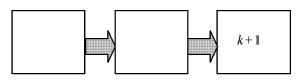图10 单方向输运 Fig.10 The single direction transport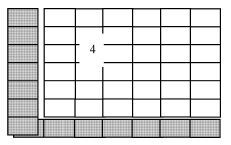图11 输运带来的关联性 Fig.11 The relevance caused by transport

 ${{u}_{k+\tfrac{1}{2}}}=\frac{1}{2}\left( {{u}_{k}}+{{u}_{k+1}} \right)$ (11)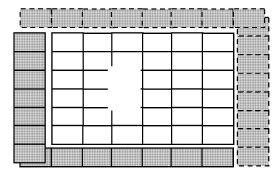图12 输运及数值格式带来的关联性 Fig.12 The relevance caused by transport and numerical format
3.2 数据相关性分析

 \begin{align} & \Delta {{V}_{ijk}}=f(\Delta t,\Delta x,\Delta y,\Delta z,{{V}_{ijk}},jfla{{g}_{ijk}},{{u}_{ijk}}, \\ & {{V}_{i\pm 1j\pm 1k\pm 1}},jfla{{g}_{i\pm 1j\pm 1k\pm 1}},{{u}_{i\pm 1j\pm 1k\pm 1}}) \\ \end{align} (12)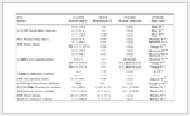表1 伪代码 Table 1 The pseudo code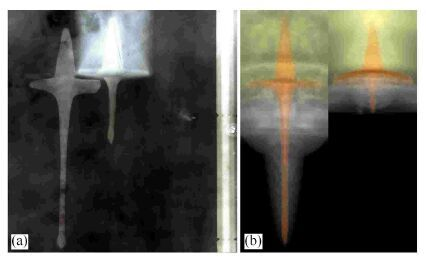图13 聚能射流数值模拟结果(b)与实验结果(a)的对比 Fig.13 The shaped jet results of numerical simulation (b) compared with experimental results (a)
4 大口径聚能装药侵彻混凝土靶的数值模拟及实验研究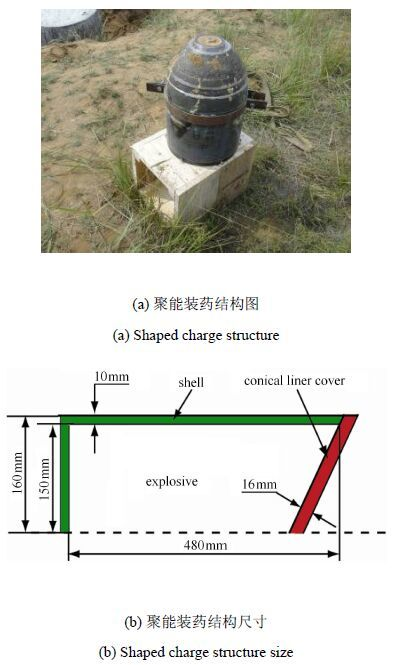图14 聚能装药结构及尺寸 Fig.14 The structure and size of shaped charge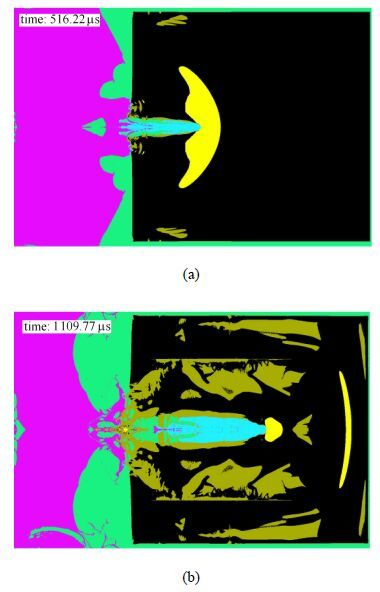图15 大口径聚能装药侵彻4 m直径混凝土靶的数值模拟结果 Fig.15 Numerical simulation results of heavy-caliber shaped charge penetration in 4 m diameter concrete target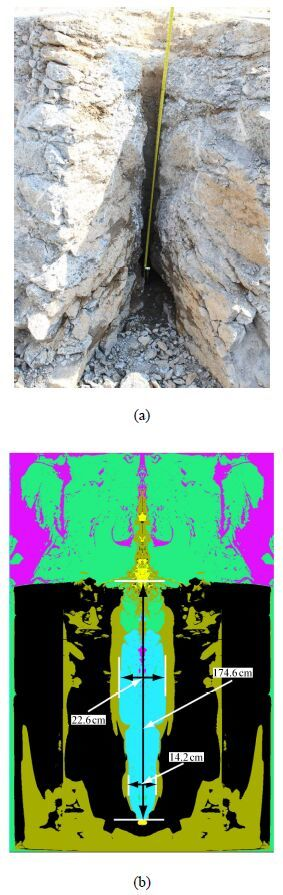图16 实验结果与数值模拟结果对比 Fig.16 Experimental results compared with numerical ones
6 结论

(1)提出了求解三维双曲守恒系统激波、接触间断等奇异性问题的伪弧长方法，数值模拟结果验证了该方法的有效性和通用性，使求解精度得到保证，该方法的提出对于提高冲击波强间断处数值模的分辨率具有重要意义；

(2)发展了一种隐-显式附加龙格-库塔算法，该算法对非刚性对流项进行显式处理，源项用半隐式格式处理，整体具有高精度和L 稳定性. 利用基元反应模型对气相爆轰问题进行了数值研究.结果表明该算法能够很好地处理源项引起的刚性问题，准确的捕捉和描述爆轰波的复杂结构和典型特征；

(3)针对爆炸与冲击问题的大规模计算需求，开展了三维多物质流体动力学欧拉数值方法特别是三维多物质界面处理算法的并行化方法研究，并对自主开发的欧拉型三维爆炸与冲击问题仿真计算程序进行了并行化设计，实现了爆炸与冲击问题的大规模并行计算；

(4)根据数值模拟得到的不同锥角的聚能装药成型规律设计了一种侵彻厚混凝土靶的聚能装药结构，并研究了靶板尺寸对聚能装药侵彻性能的影响. 对数值模拟得到的最佳混凝土靶板进行实验，实验结果表明该数值模拟技术能够有效地评估混凝土靶板的破坏情况.

  杨桂通. 计算爆炸力学及相关进展. 科学通报, 2011, 56(31): 2544-2547 (Yang Guitong. Computational explosion mechanics and related progress. Chinese Sci Bull, 2011, 56(31): 2544-2547 (in Chinese))  宁建国, 王成, 马天宝. 爆炸与冲击动力学. 北京: 国防工业出版社, 2010 (Ning Jianguo, Wang Cheng, Ma Tianbao. Explosion and Shock Dynamics. Beijing: National Defence Industry Press, 2010 (in Chinese))  宁建国, 马天宝. 计算爆炸力学. 北京: 国防工业出版社, 2015 (Ning Jianguo, Ma Tianbao. Computational Explosion Mechanics. Beijing: National Defence Industry Press, 2003 (in Chinese))  刘军, 何长江, 梁仙红. 三维弹塑性流体力学自适应欧拉方法研究. 高压物理学报, 2008, 22(1): 72-78 (Liu Jun, He Changjiang, Liang Xianhong. An Eulerian adaptive mesh refinement method for three dimensional elastic-plastic hydrodynamic simulations. Chinese Journal of High Pressure Physics, 2008, 22(1): 72-78 (in Chinese))  Wang G, Wang JT, Liu KX. New numerical algorithms in SUPER CE/SE and their applications in explosion mechanics. Science China Physics, Mechanics & Astronomy, 2010, 53(2): 237-243  Ma S, Zhang X, Lian YP, et al. Simulation of high explosive explosion using adaptive material point method. CMES: Computer Modeling in Engineering & Sciences, 2009, 39(2): 101-123  杨秀敏. 爆炸冲击现象数值模拟. 合肥: 中国科学技术大学出版社, 2010 (Yang Xiumin. Numerical Simulation for Explosion and Phenomena. Hefei: Press of University of Science and Technology of China, 2010 (in Chinese))  Ma TB,Wang C, Ning JG. Multi-material Eulerian formulations and hydrocode for the simulation of explosions. CMES: Computer Modeling in Engineering and Sciences, 2008, 33(2): 155-178  Fei GL, Ma TB, Hao L. Large-scale high performance computation on 3D explosion and shock problems. Applied Mathematics and Mechanics, 2011, 32(3): 375-382  Yang XB, Huang WZ, Qiu JX. A moving mesh WENO method for one-dimensional conservation laws. SIAM J Sci Comput, 2012,34(4): A2317-A2343  Tang HZ, Tang T. Adaptive mesh methods for one- and twodimensional hyperbolic conservation laws. SIAM J Numer Anal,2003, 41(2): 487-515  武际可, 许为厚, 丁红丽. 求解微分方程初值问题的一种弧长法. 应用数学和力学, 1999, 20(8): 875-880 (Wu Jike, Xu Weihou, Yu Hongli. Arc-length method for differential equations. Applied Mathematics and Mechanics, 1999, 20(8): 875-880 (in Chinese))  Wang X, Ma TB, Ning JG. A pseudo arc-length method for numerical simulation of shock waves. Chinese Physics Letters, 2014, 31:030201  Wang X, Ma TB, Ren HL, et al. A local pseudo arc-length method for hyperbolic conservation laws. Acta Mechanica Sinica, 2014,30(6): 956-965  Ning JG, Yuan XP, Ma TB, et al. Positivity-preserving moving mesh scheme for two-step reaction model in two dimensions. Computers and Fluids, 2015, 123: 72-86  Zhong XL. Additive semi-implicit Runge-Kutta methods for computing high-speed no equilibrium reactive flows. Journal of Computational Physics, 1996, 128(1): 19-31  Araújo AL, Murua A, Sanz-Serna JM. Symplectic methods based on decompositions. SIAM Journal of Numerical Analysis, 1997, 34(5):1926-1947  Kennedy CA, Carpenter MH. Additive Runge-Kutta schemes for convection-diffusion-reaction equations. Applied Numerical Mathematics,2003, 44(1-2): 139-181  Li J, Ren HL, Ning JG. Additive Runge-Kutta methods for H2/O2/Ar detonation with a detailed elementary chemical reaction model. Chinese Science Bulletin, 2013, 58(11): 1227  Li J, Ren HL, Ning JG. Numerical application of additive Runge- Kutta methods on detonation interaction with pipe bends. International Journal of Hydrogen Energy, 2013, 38: 9016-9027  Jiang GS, Shu CW. Efficient implementation of weighted ENO schemes. Journal of Computational Physics, 1996, 126(1): 202-228  胡湘渝, 张德良, 姜宗林. 气相爆轰基元反应模型数值模拟. 空气动力学学报, 2003, 21(1): 59-66 (Hu Xiangyu, Zhang Deliang, Jiang Zonglin. Numerical simulation of gaseous detonation with detailed chemical reaction model. Acta Aerodynamica Sinica, 2003,21(1): 59-66 (in Chinese))  Strehlow RA. Gas phase detonations: recent developments. Combustion and Flame, 1968, 12(2): 81-101  张薇, 刘云峰, 姜宗林. 气相爆轰波胞格尺度与点火延迟时间关系研究. 力学学报, 2014, 46(6): 977-981 (ZhangWei, Liu Yunfeng, Jiang Zonglin. Study on the relationship between ignition delay time and gaseous detonation cell size. Chinese Journal of Theoretical and Applied Mechanics, 2014, 46(6): 977-981 (in Chinese))  滕宏辉, 王春, 赵伟等. 斜爆轰波面上复杂结构的数值研究. 力学学报, 2011, 43(4): 641-645 (Teng Honghui, Wang Chun, Zhao Wei, et al. Numerical research on the complicated structures on the oblique detonation wave surface. Chinese Journal of Theoretical and Applied Mechanics, 2011, 43(4): 641-645 (in Chinese))  Ning JG, Ma TB, Fei GL. Multi-material Eulerian method and parallel computation for 3D explosion and impact problems. International Journal of Computational Methods, 2014, 11: 1350079  马天宝, 费广磊, 张文耀. 三维多物质弹塑性流体动力学Euler 方法的并行算法研究及程序测试. 高压物理学报, 2011, 25(6):508-513 (Ma Tianbao, Fei Guanglei, Zhang Wenyao. Study on parallel algorithm of Eulerian method for three-dimensional multimaterial plastic-elastic hydrokinetic. Chinese Journal of High Pressure Physics, 2011, 25(6): 508-513 (in Chinese))  黄风雷, 张雷雷, 段卓平. 大锥角药型罩聚能装药侵彻混凝土实验研究. 爆炸与冲击, 2008, 28(1): 17-22 (Huang Fenglei, Zhang Leilei, Duan Zhuoping. Shaped charge with large cone angle for concrete target. Explosion and Shock Waves, 2008, 28(1): 17-22 (in Chinese))  王成, 王万军, 宁建国. 聚能装药对混凝土靶板的侵彻研究. 力学学报, 2015, 47(4): 672-686 (Wang Cheng, Wang Wanjun, Ning Jianguo. Investigation on shaped charge penetration into concrete targets. Chinese Journal of Theoretical and Applied Mechanics,2015, 47(4): 672-686 (in Chinese))  许香照,马天宝,宁建国. 聚能装药侵彻混凝土数值模拟. 计算机辅助工程, 2015, 24(2): 29-35 (Xu Xiangzhao, Ma Tianbao, Ning Jianguo. Numerical simulation on concrete penetration of shaped charge. Computer Aided Engineering, 2015, 24(2): 29-35 (in Chinese)
LARGE SCALE HIGH PRECISION COMPUTATION FOR EXPLOSION AND IMPACT PROBLEMS
Ma Tianbao, Ren Huilan, Li Jian, Ning JianguoState Key Laboratory of Explosion Science and Technology, Beijing Institute of Technology, Beijing 100081, China
Abstract: Numerical simulations of explosion and impact problems have important engineering application value in the fields of national defense and civil security. Numerical simulations for these problems have a lot of di culties because the explosion and impact problems are strongly nonlinear transient dynamic problems in which multi-material mechanical behaviors are involved under the condition of high strain rate, high temperature and high pressure. Therefore, in this paper , the pseudo arc-length method for 3D nonlinear hyperbolic conservation system is proposed and the process of the algorithm realization is analyzed. The numerical results show that the algorithm improves the resolution of the shock wave strong discontinuity e ectively. The additive Runge-Kutta method for gaseous detonation numerical simulation is developed. In this method, the nonlinear convection part is solved implicitly while the chemical reaction source part is handled explicitly. The results show that the additive Runge-Kutta method can well capture and accurately describe the complex structure and typical characteristics. The parallel Eulerian numerical method of 3D multi-material hydrodynamics is investigated for the requirement of large-scale computation in engineering practical physical problems. The 3D explosion and impact problem parallel computation hydrocode is developed and the test method for this parallel hydrocode is proposed. According to the above works, some problems of large-scale and high-precision calculations for explosion and impact problems are solved. Finally, the experimental and numerical investigations on heavy-caliber shaped-charge penetration in thick concrete target are carried out, and the e ectiveness of the proposed numerical method is demonstrated by typical explosion and impact engineering problems.
Key words: explosion and impact    numerical simulation    high precision scheme    parallel computation    shaped charge jet